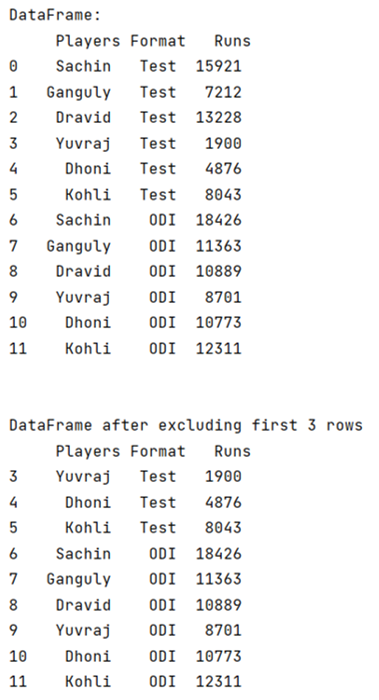# How to delete the first three rows of a DataFrame in Pandas?

Given a Pandas DataFrame, we have to delete the first three rows. By Pranit Sharma Last updated : September 21, 2023

Rows in pandas are the different cell (column) values that are aligned horizontally and also provide uniformity. Each row can have the same or different value. Rows are generally marked with the index number but in pandas, we can also assign index names according to the needs. In pandas, we can create, read, update and delete a column or row value.

## Problem statement

Given a Pandas DataFrame, we have to delete the first three rows.

## Deleting the first three rows of a DataFrame in Pandas

For this, we are going to use DataFrame.iloc[] property to directly slice the DataFrame after the first three rows. This property is used to select rows and columns by position/index.

Note

To work with pandas, we need to import pandas package first, below is the syntax:

```import pandas as pd
```

Let us understand with the help of an example.

## Python program to delete the first three rows of a DataFrame in Pandas

```# Importing pandas package
import pandas as pd

# creating a dictionary of student marks
d = {
"Players":['Sachin','Ganguly','Dravid','Yuvraj','Dhoni','Kohli',
'Sachin','Ganguly','Dravid','Yuvraj','Dhoni','Kohli'],
"Format":['Test','Test','Test','Test','Test','Test',
'ODI','ODI','ODI','ODI','ODI','ODI'],
"Runs":[15921,7212,13228,1900,4876,8043,
18426,11363,10889,8701,10773,12311]}

# Now we will create DataFrame
df = pd.DataFrame(d)

# Viewing the DataFrame
print("DataFrame:\n",df,"\n\n")

# Excluding first three rows
result = df.iloc[3:]

# Display resulted dataframe
print("DataFrame after excluding first 3 rows\n",result)
```

### Output

The output of the above program is: